### DIGITAL Math Lessons for Distance LearningWhy I love using PowerPoint lessons to teach math?
I have created and used math PowerPoint presentations with my middle school students for the past 17 years, and I am excited to share them with you. These lessons are perfect for distance learning or in-person lessons.

🕑Use Teaching Time More Efficiently
It takes less time to begin a lesson because each digital lesson is saved to my computer. I click on the presentation and I am ready to begin.

🕑Accommodate Different Learning Styles
Using these PowerPoint math lessons allow me to effectively teach in different styles to my students. There are students who are more verbally inclined, so they can listen to me talk. My visual learners can clearly see the engaging diagrams that I have included for each math concept.

What is included in each lesson?

✅Mini-Lesson with Guided Practice
The mini-lesson for each lesson includes essential vocabulary and key terms for that topic. Students are then guided through scaffolded instruction with guided practice questions for each lesson objective. All of the math problems are worked out step-by-step with detailed explanations.

Lesson Quiz
Every lesson ends with a lesson quiz that includes questions from each topic of this lesson. This is perfect for assessing your students' understanding of this lesson. I have students complete these questions individually or with a partner.

Exit Ticket
I have included an exit ticket question at the end of each presentation. Students write the answer on a post-it and turn it into me on their way out the door.  If you are teaching remote they can post their answers to the exit ticket using their devices.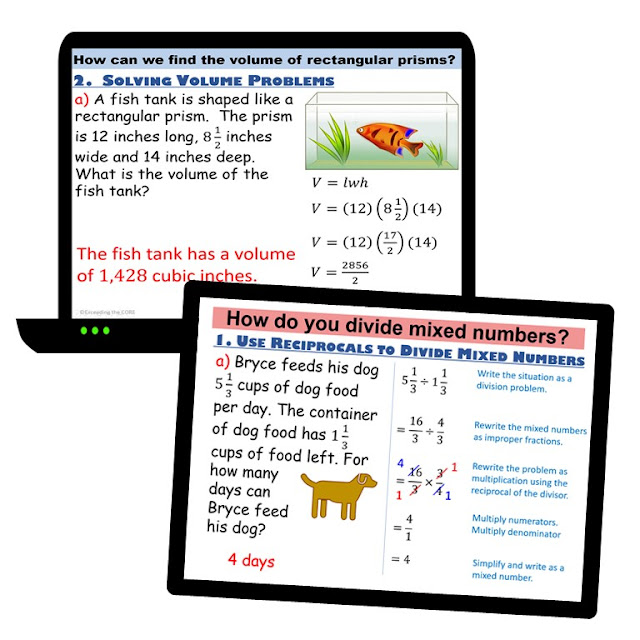Math PowerPoint Lessons & Guided Practice

As of now, I have made PowerPoint math lessons for the following grades and topics ( check them out!!). If you do not see the math topic you want please check my Blog Store or my  TpT Store.  I add new resources every week!

##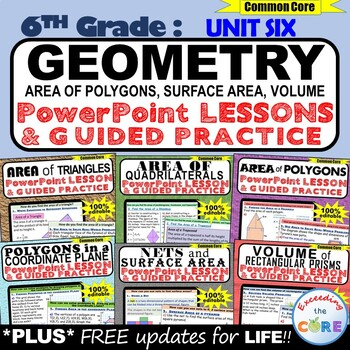Geometry Unit: 6th Grade PowerPoint Lessons & Practice Bundle Area of QuadrilateralsArea of TrianglesArea of PolygonsPolygons in the Coordinate PlaneNets and Surface AreaVolume of Rectangular Prisms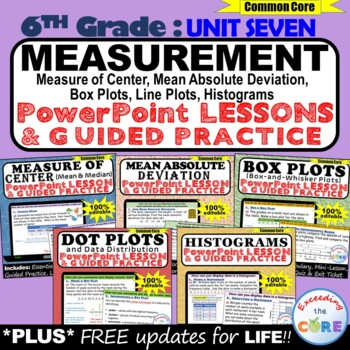Measurement and Data: 6th Gade PowerPoint Lessons & Practice BundleMeasure of Center (mean and median)Mean Absolute Deviation (MAD)Box Plots (Box-and-Whisker Plots)Dot Plots (Line Plots) and Data DistributionHistograms and Frequency Tables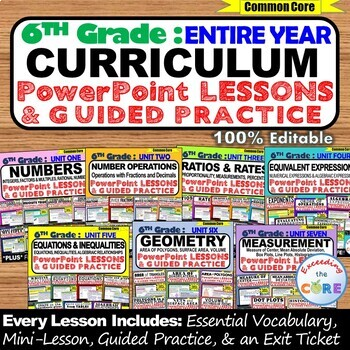6th Grade CURRICULUM PowerPoint Lessons & Guided Practice Bundle (ENTIRE YEAR)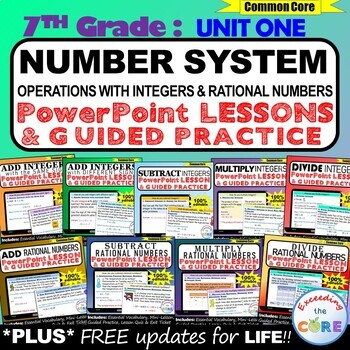Number System: 7th Grade PowerPoint Lessons & Guided Practice BundleAdd Integers with the Same SignAdd Integers with Different SignsSubtract IntegersMultiply IntegersDivide IntegersAdd Rational NumbersSubtract Rational NumbersMultiply Rational NumbersDivide Rational Numbers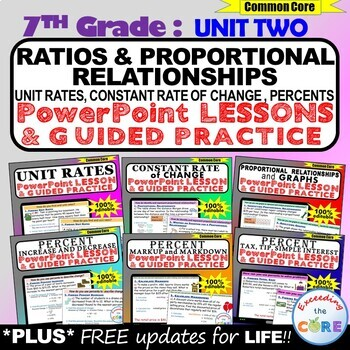Ratios & Proportional Relationships: 6th Grade PowerPoint Lessons Guided Practice BundleUnit RatesConstant Rates of ChangeProportional Relationships and GraphsPercent - Increase and DecreasePercent - Markups and MarkdownsPercent - Sales Tax, Tip and Simple Interest

 Expressions, Equations, Inequalites: 7th Grade PowerPoint Lessons Guided Practice Bundle